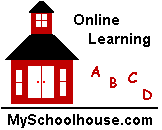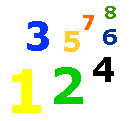# Telling Time

Telling Time Worksheet - Elementary Lessons & Worksheets on Telling Time - My Schoolhouse - Online Learning

Learn to tell time to the hour and minute by reading the minute hand and hour hand on the clocks below.

A clock has numbers and hands.

The long hand is called the minute hand.

The short hand is called the hour hand.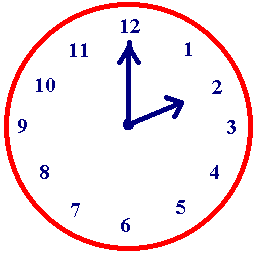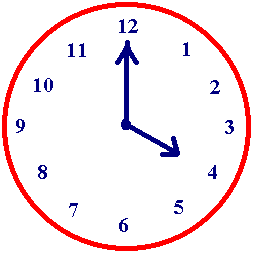The long hand is pointing to the 12.The short hand is pointing to the 2. This clock shows 2 o'clock. The long hand is pointing to the 12.The short hand is pointing to the 4. This clock shows 4 o'clock.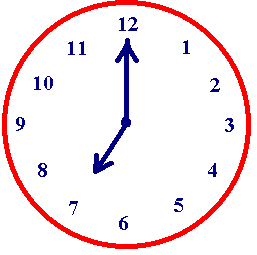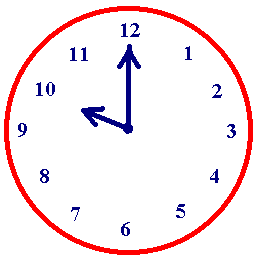The long hand is pointing to the 12.The short hand is pointing to the 7. This clock shows o'clock. The long hand is pointing to the 12.The short hand is pointing to the 10. This clock shows o'clock.

Write the time for each clock below.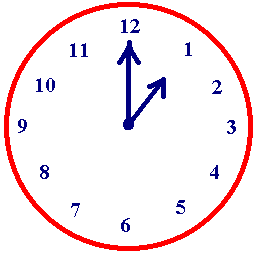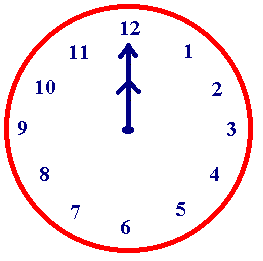o'clock o'clock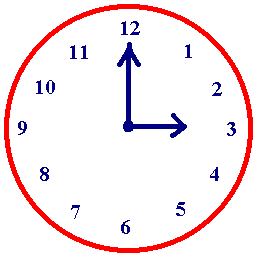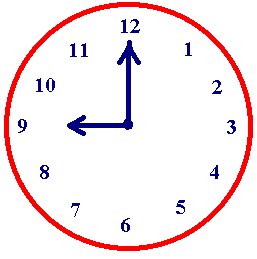o'clock o'clock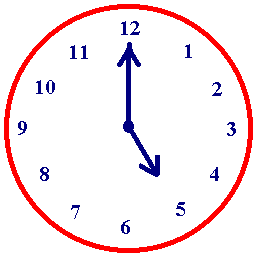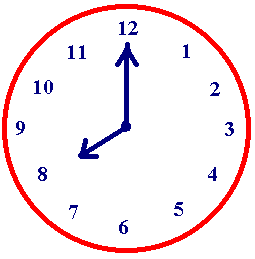o'clock o'clock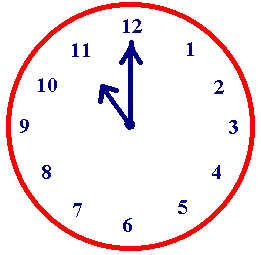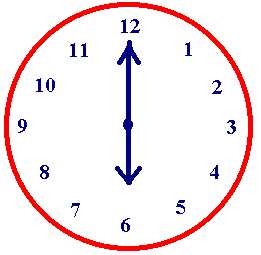o'clock o'clock

 Thank You for using My Schoolhouse